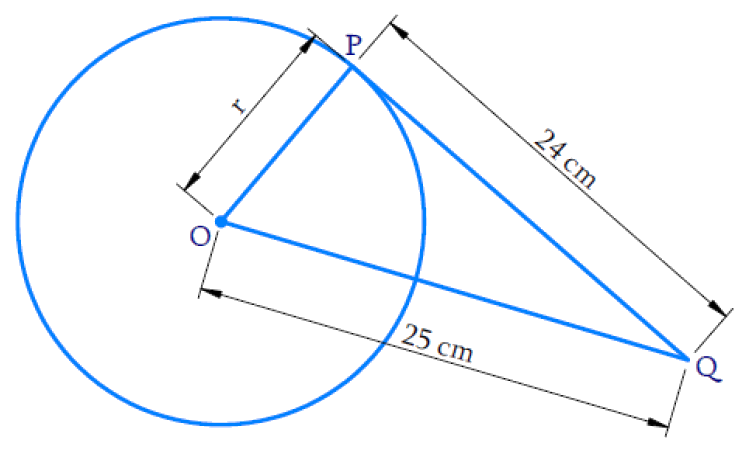# Ex.10.2 Q1 Circles Solution - NCERT Maths Class 10

Go back to  'Ex.10.2'

## Question

From a point $$Q,$$ the length of the tangent to a circle is $$\text{24 cm}$$ and the distance of $$Q$$ from the center is $$\text{25 cm.}$$

The radius of the circle is

(A)    $$\text{7 cm}$$

(B)     $$\text{12 cm}$$

(C)     $$\text{15 cm}$$

(D)     $$\text{24.5 cm}$$

Video Solution
Circles
Ex 10.2 | Question 1

## Text Solution

What is Known?

(i) Length of tangent from a point $$Q,$$ i.e. $$PQ$$ is $$\text{24 cm}$$

(ii) Distance of $$Q$$ from center, i.e. $$OQ$$ is $$\text{25 cm.}$$What is Unknown?

Reasoning:

Tangent at any point of a circle is perpendicular to the radius through the point of contact.

Steps:

$$\therefore \; \Delta \,{OPQ}$$ is a right-angled triangle

By Pythagoras theorem,

\begin{align} {O Q} ^ { 2 } &= {O P} ^ { 2 } + {P Q} ^ { 2 } \\ 25 ^ { 2 } &= r ^ { 2 } + 24 ^ { 2 } \\ r ^ { 2 } &= 25 ^ { 2 } - 24 ^ { 2 } \\ & = 625 - 576 \\ r ^ { 2 } &= 49 \\ r& = \pm 7 \end{align}

Radius cannot be a negative value,

$$\therefore\; r = + 7 \; \rm{cm}$$

Hence the correct  Option is A

Learn from the best math teachers and top your exams

• Live one on one classroom and doubt clearing
• Practice worksheets in and after class for conceptual clarity
• Personalized curriculum to keep up with school